Publicité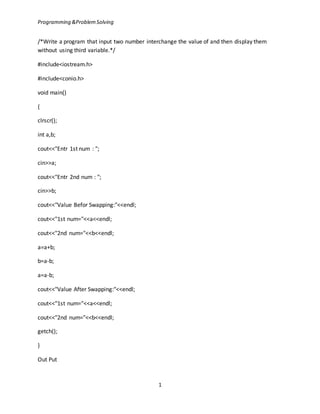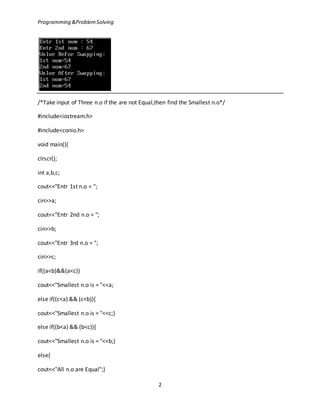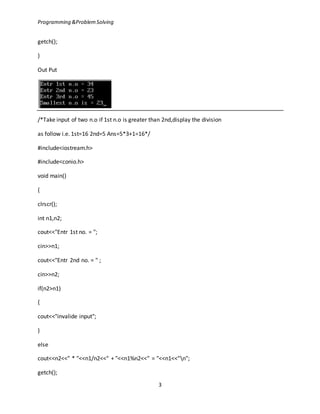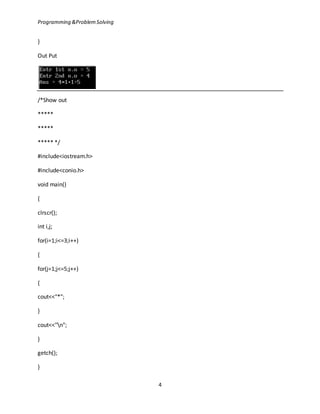Publicité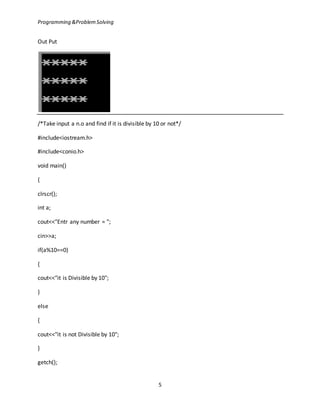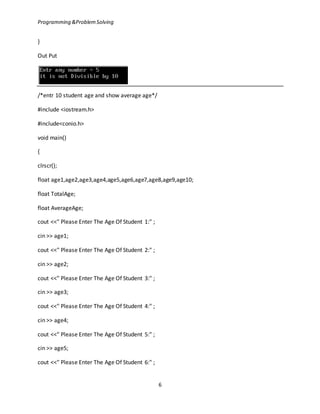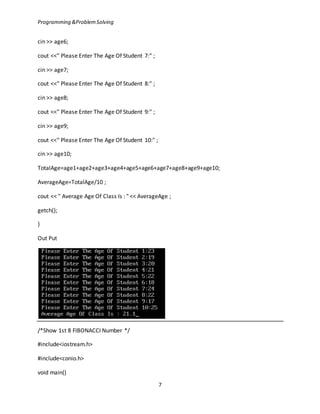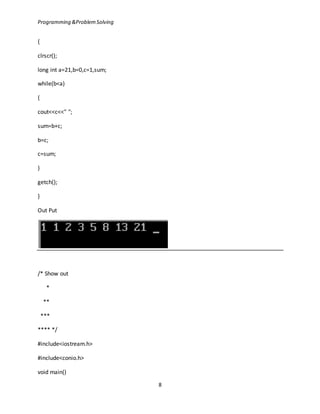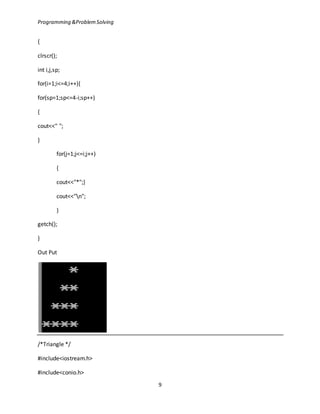Publicité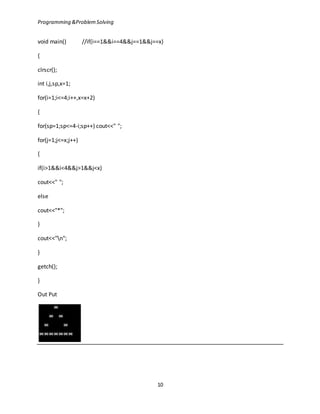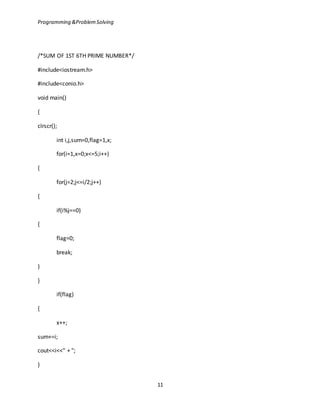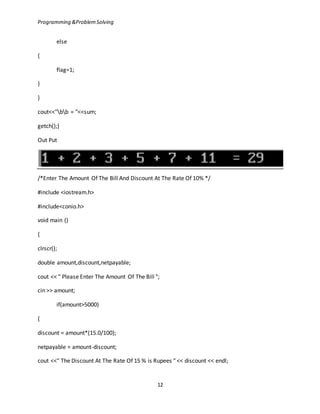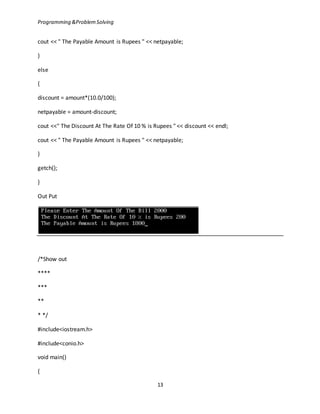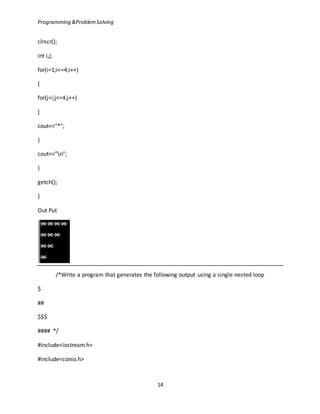Publicité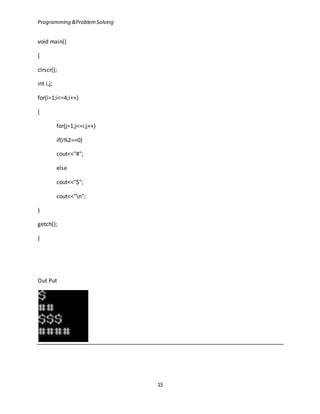Prochain SlideShare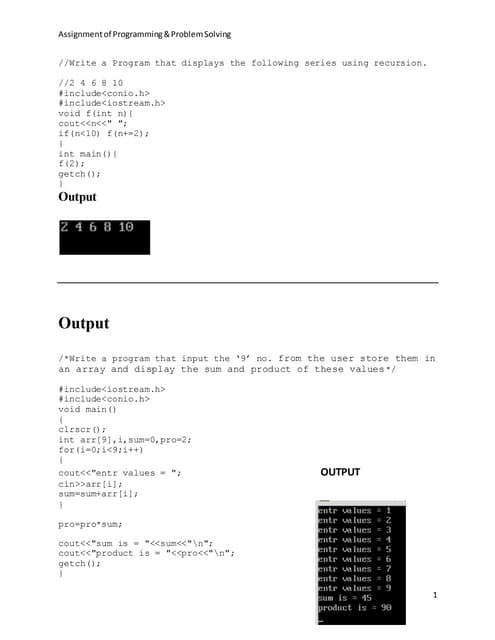Assignement of programming & problem solving ass.(3)
Chargement dans ... 3
1 sur 15
Publicité

### Assignement of programming & problem solving

1. Programming &ProblemSolving 1 /*Write a program that input two number interchange the value of and then display them without using third variable.*/ #include<iostream.h> #include<conio.h> void main() { clrscr(); int a,b; cout<<"Entr 1st num : "; cin>>a; cout<<"Entr 2nd num : "; cin>>b; cout<<"Value Befor Swapping:"<<endl; cout<<"1st num="<<a<<endl; cout<<"2nd num="<<b<<endl; a=a+b; b=a-b; a=a-b; cout<<"Value After Swapping:"<<endl; cout<<"1st num="<<a<<endl; cout<<"2nd num="<<b<<endl; getch(); } Out Put
2. Programming &ProblemSolving 2 /*Take input of Three n.o if the are not Equal,then find the Smallest n.o*/ #include<iostream.h> #include<conio.h> void main(){ clrscr(); int a,b,c; cout<<"Entr 1st n.o = "; cin>>a; cout<<"Entr 2nd n.o = "; cin>>b; cout<<"Entr 3rd n.o = "; cin>>c; if((a<b)&&(a<c)) cout<<"Smallest n.o is = "<<a; else if((c<a) && (c<b)){ cout<<"Smallest n.o is = "<<c;} else if((b<a) && (b<c)){ cout<<"Smallest n.o is = "<<b;} else{ cout<<"All n.o are Equal";}
3. Programming &ProblemSolving 3 getch(); } Out Put /*Take input of two n.o if 1st n.o is greater than 2nd,display the division as follow i.e. 1st=16 2nd=5 Ans=5*3+1=16*/ #include<iostream.h> #include<conio.h> void main() { clrscr(); int n1,n2; cout<<"Entr 1st no. = "; cin>>n1; cout<<"Entr 2nd no. = " ; cin>>n2; if(n2>n1) { cout<<"invalide input"; } else cout<<n2<<" * "<<n1/n2<<" + "<<n1%n2<<" = "<<n1<<"n"; getch();
4. Programming &ProblemSolving 4 } Out Put /*Show out ***** ***** ***** */ #include<iostream.h> #include<conio.h> void main() { clrscr(); int i,j; for(i=1;i<=3;i++) { for(j=1;j<=5;j++) { cout<<"*"; } cout<<"n"; } getch(); }
5. Programming &ProblemSolving 5 Out Put /*Take input a n.o and find if it is divisible by 10 or not*/ #include<iostream.h> #include<conio.h> void main() { clrscr(); int a; cout<<"Entr any number = "; cin>>a; if(a%10==0) { cout<<"it is Divisible by 10"; } else { cout<<"it is not Divisible by 10"; } getch();
6. Programming &ProblemSolving 6 } Out Put /*entr 10 student age and show average age*/ #include <iostream.h> #include<conio.h> void main() { clrscr(); float age1,age2,age3,age4,age5,age6,age7,age8,age9,age10; float TotalAge; float AverageAge; cout <<" Please Enter The Age Of Student 1:" ; cin >> age1; cout <<" Please Enter The Age Of Student 2:" ; cin >> age2; cout <<" Please Enter The Age Of Student 3:" ; cin >> age3; cout <<" Please Enter The Age Of Student 4:" ; cin >> age4; cout <<" Please Enter The Age Of Student 5:" ; cin >> age5; cout <<" Please Enter The Age Of Student 6:" ;
7. Programming &ProblemSolving 7 cin >> age6; cout <<" Please Enter The Age Of Student 7:" ; cin >> age7; cout <<" Please Enter The Age Of Student 8:" ; cin >> age8; cout <<" Please Enter The Age Of Student 9:" ; cin >> age9; cout <<" Please Enter The Age Of Student 10:" ; cin >> age10; TotalAge=age1+age2+age3+age4+age5+age6+age7+age8+age9+age10; AverageAge=TotalAge/10 ; cout << " Average Age Of Class Is : " << AverageAge ; getch(); } Out Put /*Show 1st 8 FIBONACCI Number */ #include<iostream.h> #include<conio.h> void main()
8. Programming &ProblemSolving 8 { clrscr(); long int a=21,b=0,c=1,sum; while(b<a) { cout<<c<<" "; sum=b+c; b=c; c=sum; } getch(); } Out Put /* Show out * ** *** **** */ #include<iostream.h> #include<conio.h> void main()
9. Programming &ProblemSolving 9 { clrscr(); int i,j,sp; for(i=1;i<=4;i++){ for(sp=1;sp<=4-i;sp++) { cout<<" "; } for(j=1;j<=i;j++) { cout<<"*";} cout<<"n"; } getch(); } Out Put /*Triangle */ #include<iostream.h> #include<conio.h>
10. Programming &ProblemSolving 10 void main() //if(i==1&&i==4&&j==1&&j==x) { clrscr(); int i,j,sp,x=1; for(i=1;i<=4;i++,x=x+2) { for(sp=1;sp<=4-i;sp++) cout<<" "; for(j=1;j<=x;j++) { if(i>1&&i<4&&j>1&&j<x) cout<<" "; else cout<<"*"; } cout<<"n"; } getch(); } Out Put
11. Programming &ProblemSolving 11 /*SUM OF 1ST 6TH PRIME NUMBER*/ #include<iostream.h> #include<conio.h> void main() { clrscr(); int i,j,sum=0,flag=1,x; for(i=1,x=0;x<=5;i++) { for(j=2;j<=i/2;j++) { if(i%j==0) { flag=0; break; } } if(flag) { x++; sum+=i; cout<<i<<" + "; }
12. Programming &ProblemSolving 12 else { flag=1; } } cout<<"bb = "<<sum; getch();} Out Put /*Enter The Amount Of The Bill And Discount At The Rate Of 10% */ #include <iostream.h> #include<conio.h> void main () { clrscr(); double amount,discount,netpayable; cout << " Please Enter The Amount Of The Bill "; cin >> amount; if(amount>5000) { discount = amount*(15.0/100); netpayable = amount-discount; cout <<" The Discount At The Rate Of 15 % is Rupees " << discount << endl;
13. Programming &ProblemSolving 13 cout << " The Payable Amount is Rupees " << netpayable; } else { discount = amount*(10.0/100); netpayable = amount-discount; cout <<" The Discount At The Rate Of 10 % is Rupees " << discount << endl; cout << " The Payable Amount is Rupees " << netpayable; } getch(); } Out Put /*Show out **** *** ** * */ #include<iostream.h> #include<conio.h> void main() {
14. Programming &ProblemSolving 14 clrscr(); int i,j; for(i=1;i<=4;i++) { for(j=i;j<=4;j++) { cout<<"*"; } cout<<"n"; } getch(); } Out Put /*Write a program that generates the following output using a single nested loop \$ ## \$\$\$ #### */ #include<iostream.h> #include<conio.h>
15. Programming &ProblemSolving 15 void main() { clrscr(); int i,j; for(i=1;i<=4;i++) { for(j=1;j<=i;j++) if(i%2==0) cout<<"#"; else cout<<"\$"; cout<<"n"; } getch(); } Out Put
Publicité